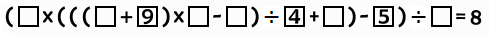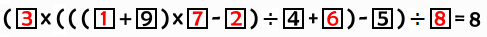## 算式填空數學謎題#### 解析

(A×(((B+9)×C-D)÷4+E)-5)÷F=8，

1. F=1，(8×F+5)=13，沒有合理的A。
2. F=2，(8×F+5)=21，A是1或3或7。
3. F=3，(8×F+5)=29，A是1。
4. F=6，(8×F+5)=53，沒有合理的A。
5. F=7，(8×F+5)=61，沒有合理的A。
6. F=8，(8×F+5)=69，A是3。

• 如果F=2,A=1，要用3,6,7,8湊出((B+9)×C-D)÷4+E=21。
1. B=3,C=6，選D=8,E=7，但((3+9)×6-8)÷4+7=23。
2. B=6,C=3，無法整除。
3. B=3,C=7，選D=8,E=6，但((3+9)×7-8)÷4+6=25。
4. B=7,C=3，選D=8,E=6，但((7+9)×3-8)÷4+6=16。
5. B=3,C=8，無法整除。
6. B=8,C=3，選D=7,E=6，但((8+9)×3-7)÷4+6=17。
7. B=6,C=7，無法整除。
8. B=7,C=6，選D=8,E=3，但((7+9)×6-8)÷4+3=25。
9. B=6,C=8，無法整除。
10. B=8,C=6，無法整除。
11. B=7,C=8，無法整除。
12. B=8,C=7，選D=3,E=6，但((8+9)×7-3)÷4+6=35。
• 如果F=2,A=3，要用1,6,7,8湊出((B+9)×C-D)÷4+E=7。
盡可能安排到算出最小，((7+9)×1-8)÷4+6=8也還是超過了7。
• 如果F=2,A=7，要用1,3,6,8湊出((B+9)×C-D)÷4+E=3。
盡可能安排到算出最小，((6+9)×1-8)÷4+3=4.75也還是超過了3。
• 如果F=3，A=1，要用2,6,7,8湊出((B+9)×C-D)÷4+E=29。
1. B=2,C=6，無法整除。
2. B=6,C=2，無法整除。
3. B=2,C=7，無法整除。
4. B=7,C=2，選D=8,E=6，但((7+9)×2-8)÷4+6=12。
5. B=2,C=8，無法整除。
6. B=8,C=2，選D=6,E=7，但((8+9)×2-6)÷4+7=14。
7. B=6,C=7，無法整除。
8. B=7,C=6，選D=8,E=2，但((7+9)×6-8)÷4+2=24。
9. B=6,C=8，無法整除。
10. B=8,C=6，選D=2,E=7，但((8+9)×6-2)÷4+7=32。
11. B=7,C=8，無法整除。
12. B=8,C=7，無法整除。
• 如果F=8,A=3，要用1,2,6,7湊出((B+9)×C-D)÷4+E=23。
1. B=1,C=2，無法整除。
2. B=2,C=1，選D=7,E=6，但((2+9)×1-7)÷4+6=7。
3. B=1,C=6，無法整除。
4. B=6,C=1，選D=7,E=2，但((6+9)×1-7)÷4+2=4。
5. B=1,C=7，選D=6,E=2，但((1+9)×7-6)÷4+2=18；
不過也能選D=2,E=6，正好((1+9)×7-2)÷4+6=23。
6. B=7,C=1，無法整除。
7. B=2,C=6，無法整除。
8. B=6,C=2，無法整除。
9. B=2,C=7，選D=1,E=6，但((2+9)×7-1)÷4+6=25。
10. B=7,C=2，無法整除。
11. B=6,C=7，選D=1,E=2，但((6+9)×7-1)÷4+2=28。
12. B=7,C=6，無法整除。

8,392